# ISRO Scientist or Engineer Electronics Dec 2017

Instructions

For the following questions answer them individually

Question 1

# Find the area of the region bounded by the curves $$y = x^{2}, y = \frac{1}{x}$$ and $$x = \frac{1}{2}$$ (see in graphical representive figure)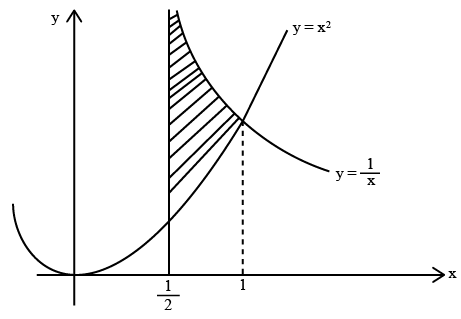Question 2

# Find the Eigen values $$\lambda$$ in the system $$\begin{bmatrix}4 & 1 \\3 & 2 \end{bmatrix}$$ $$\begin{bmatrix} x \\ y \end{bmatrix}$$ =\lambda $$\begin{bmatrix} x \\ y \end{bmatrix}$$Question 3

# Find the transient response of a pair of complex poles as given below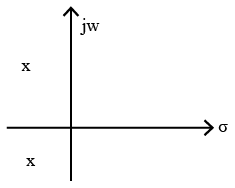Question 4

# A random variable z , has a probability density function f(z) where $$f(Z) =e^{-z} O\leq Z < \infty$$ the probability of $$O \leq Z \leq 2$$ will be approximatelyQuestion 5

# Evaluate $$\lim_{x \rightarrow 2} \frac{\sqrt{x^{2}+5-3}}{x^{2}-2x}$$Question 6

# What is the R.M.S of following waveform if the average value is zero?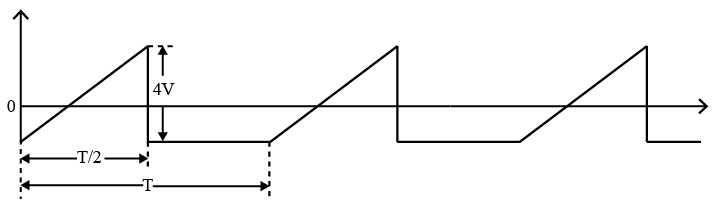Question 7

# A radar system uses TWTA as high power RF source for transmitting 300W Peak Power. The efficiency of transmitter during pulse is 75% and the transmit duty is 25%. If the DC power required during pulse off period is 20W. The average power dissipation in TWTA isQuestion 8

# The ramp signal $$(V_{t} : 0 to 5V)$$ is compared with the Soft-Start Signal provided by N-channel MOSFET $$(Q_{1} )$$ for Amplifier $$( A_{1} )$$ output. If $$Q_{1}$$ having low threshold voltage of 0.7V and 1 negligible ON resistance. What is the duty of $$c_{1}$$ output signal of comparator $$(C _{1})$$ after $$100 \mu$$ Sec?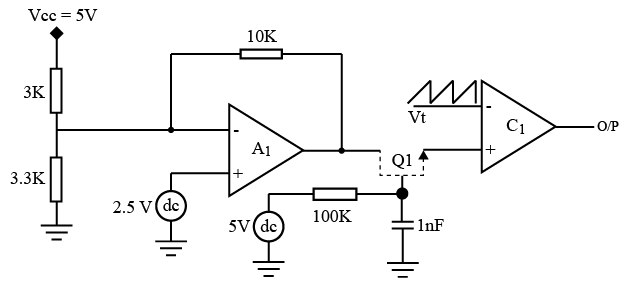Question 9

# A High speed digital Subsystem requires three voltages $$V_{1} , V_{2}$$ and $$V_{3}$$ with 1 : 2 : 1 power 3 ratings respectively. The power supply is designed with the distribute power conversion scheme as shown in the following figure. What is the overall power conversion efficiency?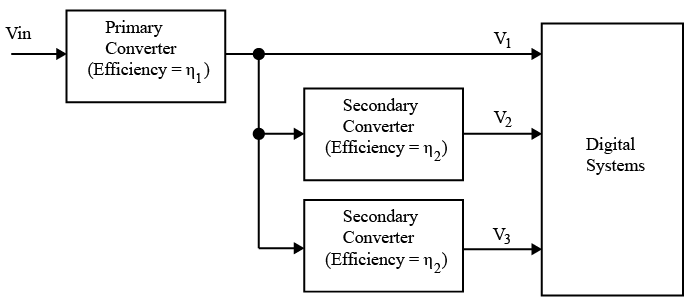Question 10

# If the transformer and diodes in the following circuit are ideal, Find out the value of Capacitor (Co) provides 5% ripple voltage across $$R_{L}$$. (Assume that $$\sin^{-1} (0.95) \sim \frac{2 \pi}{5}$$ in Radians $$\ln(0.95) = -0.051$$)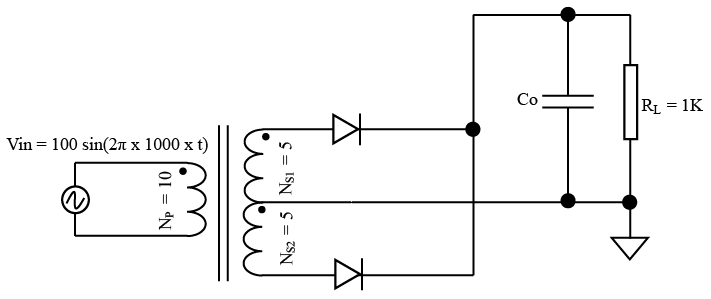OR